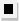Chapter 13.6, Problem 50E### Algebra and Trigonometry (MindTap ...

4th Edition
James Stewart + 2 others
ISBN: 9781305071742

#### Solutions

Chapter
Section### Algebra and Trigonometry (MindTap ...

4th Edition
James Stewart + 2 others
ISBN: 9781305071742
Textbook Problem

# 4 9 - 5 2Proving a statement Show that the given statement is true. ( n 0 ) = 1 and ( n n ) = 1

To determine

To prove:

(n0)=1 and (nn)=1

Explanation

Approach:

Use the formula for the binomial coefficient.

Calculation:

(n0)=n!0!(n0)!=n!n!=1

### Still sussing out bartleby?

Check out a sample textbook solution.

See a sample solution

#### The Solution to Your Study Problems

Bartleby provides explanations to thousands of textbook problems written by our experts, many with advanced degrees!

Get Started